## Applications

#### Chapter index in this window —   — Chapter index in separate window

This material (including images) is copyrighted!. See my copyright notice for fair use practices.

### 1) Kepler's Second Law of orbital motion

The area swept out by a line connecting an orbiting object and the central point is the same for any two equal periods of times. That line is called a radius vector in the following discussion.

The rate of change of the swept-out area does NOT change with time. The line along which gravity acts is parallel to the radius vector. This means that there are no torques disturbing the angular motion and, therefore, angular momentum is conserved. The part of the orbital velocity (v-orbit) perpendicular (at a right angle) to the radius vector (r) is vt. The rate of change of the swept-out area = r×vt/2.

To calculate the orbital angular momentum use vt for the velocity. So, the angular momentum = mass × vt × r = mass × 2 × (rate of change of area). That value does not change over time. So if r decreases, v-orbit (and vt) must increase! If r increases, v-orbit (and vt) must decrease. This is just what Kepler observed for the planets!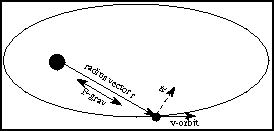### 2) Earth-Moon system

The total angular momentum = spin angular momentum + orbital angular momentum. The total angular momentum is CONSTANT. To find the spin angular momentum, subdivide the object into small pieces of mass and find the angular momentum for each of the small pieces. Then add up the angular momentum for all of the pieces. The Earth's spin speed is decreasing so its spin angular momentum is DEcreasing. Therefore, the Moon's orbital angular momentum must compensate by INcreasing. It does this by increasing the Earth-Moon distance.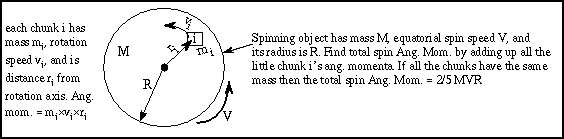### 3) Rapidly spinning neutron stars

Originally, a big star has a core 10,000's to 100,000's kilometers in radius (the whole star is even bigger!). Here the radius is used instead of the diameter, because what is important is how far each piece of the core is from the spin axis that goes through the exact center of the star.

The core spins at 2 to 10 kilometers/second at the core's equator. If no external forces produce torques, the angular momentum is constant. During a supernova the outer layers are blown off and the core shrinks to only 10 kilometers in radius! The core angular momentum is approximately = 0.4×M×V×R and the mass M has stayed approximately the same. When the radius R shrinks by factors of 10,000's, the spin speed V must increase by 10,000's of times.

Sometimes the neutron star suddenly shrinks slightly (by a millimeter or so) and it spins faster. The neutron star produces radiation from its strong magnetic field. This radiation is produced at the expense of the rotational energy and the angular momentum is not strictly conserved---it slowly decreases. Therefore, the neutron star spin speed slowly decreases.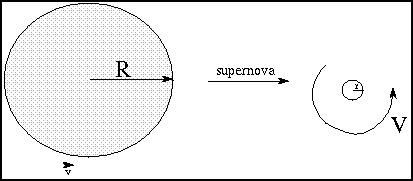### 4) Accretion disk in a binary system

Gas flowing from one star falls toward its compact companion into an orbit around it. The orbital angular momentum is conserved, so as the gas' distance from the compact companion DEcreases, its orbital speed must INcrease. It forms a rapidly rotating disk-like whirlpool called an accretion disk. Over time some of the gas in the disk gas give torques to other parts of the disk's orbital motions through friction. This causes their angular momentum to decrease. Some of that gas, then, eventually falls onto the compact companion.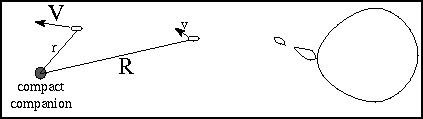### 5) Forming Galaxy

A huge slowly spinning gas cloud collapses. Parts of the roughly spherical gas cloud break up into small chunks to form stars and globular clusters. As the rest of the gas cloud collapses, the inner, denser parts collapse more rapidly than the less dense parts. Stars form in the inner, denser parts before they form in the outer, less dense parts.

All the time as the cloud collapses, the spin speed must increase. Since no outside forces produce torques, the angular momentum is conserved. The rapidly spinning part of gas cloud eventually forms a disk. This is because the cloud can collapse more easily in a direction parallel to the spin axis. The gas that is orbiting perpendicular to the spin axis has enough inertia to resist the inward pull of gravity (the gas feels a ``centrifugal force''). The densest parts of the disk will form stars.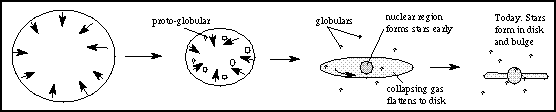#### Go to Astronomy Notes home

last updated: 28 May 2001